# Learning Hierarchy-Aware Knowledge Graph Embeddings for Link Prediction

## Basic Idea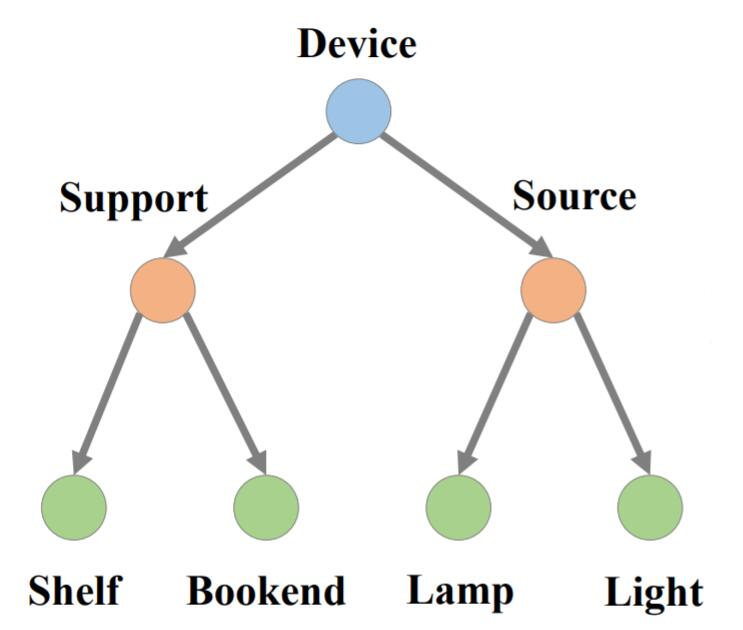$$[\mathbf{a} \circ \mathbf{b}]_{i}=[\mathbf{a}]_{i} \cdot[\mathbf{b}]_{i}$$

$[\mathbf{h}]_i$ 代表第$i$ 个实体的Embedding, $\lVert\cdot\rVert_1$, $\lVert \cdot \rVert_2$ 分别代表L1范数和L2范数.

## HAKE

• 模长部分对不同层级的实体建模.
• 角度部分对同一层级的实体建模.

• 模长部分对应着固定角度下的放缩.
• 角度部分对应着固定模长时的旋转.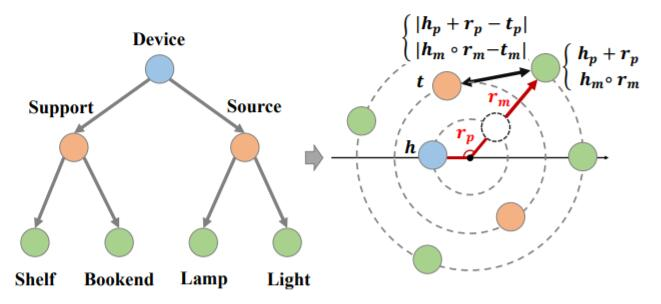### Hierarchy - Aware Knowledge Graph Embedding

#### Modulus Part

$$\mathbf{h}_{m} \circ \mathbf{r}_{m}=\mathbf{t}_{m}, \text { where } \mathbf{h}_{m}, \mathbf{t}_{m} \in \mathbb{R}^{k}, \text { and } \mathbf{r}_{m} \in \mathbb{R}_{+}^{k}$$

$$d_{r, m}\left(\mathbf{h}_{m}, \mathbf{t}_{m}\right)=\lVert\mathbf{h}_{m} \circ \mathbf{r}_{m}-\mathbf{t}_{m}\rVert_{2}$$

• 对于正样本, $[\mathbf{h}]_i, [\mathbf{t}_1]_i$ 倾向于使用同一个符号, 因为$[\mathbf{r}_m]_i>0$.
• 对于负例, $[\mathbf{h}_m]_i, [\mathbf{t}_{2,m}]_i$ 可能是随机的符号.

#### Phrase Part

$$\mathbf{h}_p+\mathbf{r}_p \approx\mathbf{t}_p$$

$$\left(\mathbf{h}_{p}+\mathbf{r}_{p}\right) \bmod 2 \pi=\mathbf{t}_{p}, \text { where } \mathbf{h}_{p}, \mathbf{r}_{p}, \mathbf{t}_{p} \in[0,2 \pi)^{k}$$

$$d_{r, p}\left(\mathbf{h}_{p}, \mathbf{t}_{p}\right)=\lVert\sin \left(\left(\mathbf{h}_{p}+\mathbf{r}_{p}-\mathbf{t}_{p}\right) / 2\right)\rVert_{1}$$

#### Map into Polar Coordinate System

$$\left\{\begin{array}{l} \mathbf{h}_{m} \circ \mathbf{r}_{m}=\mathbf{t}_{m}, \text { where } \mathbf{h}_{m}, \mathbf{t}_{m} \in \mathbb{R}^{k}, \mathbf{r}_{m} \in \mathbb{R}_{+}^{k} \\ \left(\mathbf{h}_{p}+\mathbf{r}_{p}\right) \bmod 2 \pi=\mathbf{t}_{p}, \text { where } \mathbf{h}_{p}, \mathbf{t}_{p}, \mathbf{r}_{p} \in[0,2 \pi)^{k} \end{array}\right.$$

$$d_{r}(\mathbf{h}, \mathbf{t})=d_{r, m}\left(\mathbf{h}_{m}, \mathbf{t}_{m}\right)+\lambda d_{r, p}\left(\mathbf{h}_{p}, \mathbf{t}_{p}\right)$$

HAKE的打分函数如下:

$$f_{r}(\mathbf{h}, \mathbf{t})=-d_{r}(\mathbf{h}, \mathbf{t})=-d_{r, m}(\mathbf{h}, \mathbf{t})-\lambda d_{r, p}(\mathbf{h}, \mathbf{t})$$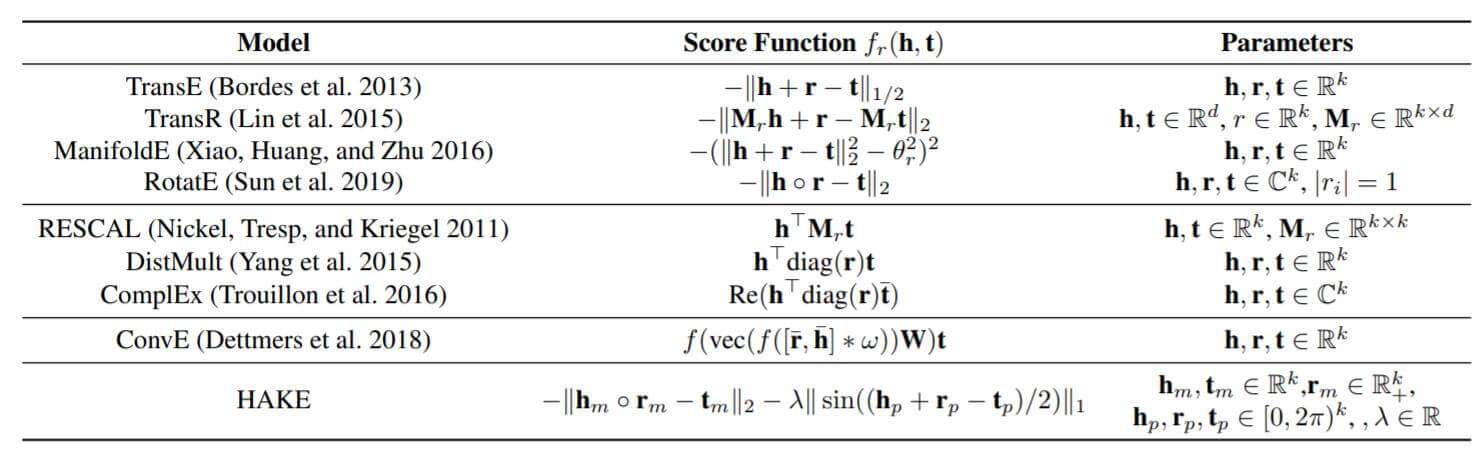$$d_{r, m}^{\prime}(\mathbf{h}, \mathbf{t})=\lVert\mathbf{h}_{m} \circ \mathbf{r}_{m}+\left(\mathbf{h}_{m}+\mathbf{t}_{m}\right) \circ \mathbf{r}_{m}^{\prime}-\mathbf{t}_{m}\rVert_{2}$$

$$d_{r, m}^{\prime}(\mathbf{h}, \mathbf{t})=\lVert\mathbf{h}_{m} \circ\left(\left(\mathbf{r}_{m}+\mathbf{r}_{m}^{\prime}\right) /\left(1-\mathbf{r}_{m}^{\prime}\right)\right)-\mathbf{t}_{m}\rVert_{2}$$

### Loss Function

HAKE与RotatE一样, 使用最大化间隔的损失函数来优化:

$$L=-\log \sigma\left(\gamma-d_{r}(\mathbf{h}, \mathbf{t})\right) -\sum_{i=1}^{n} p\left(h_{i}^{\prime}, r, t_{i}^{\prime}\right) \log \sigma\left(d_{r}\left(\mathbf{h}_{i}^{\prime}, \mathbf{t}_{i}^{\prime}\right)-\gamma\right)$$

HAKE也使用了RotatE中提到的自对抗负采样, 我在RotatE中已经有过讲解:
$$p\left(h_{j}^{\prime}, r, t_{j}^{\prime} \mid\left\{\left(h_{i}, r_{i}, t_{i}\right)\right\}\right)=\frac{\exp \alpha f_{r}\left(\mathbf{h}_{j}^{\prime}, \mathbf{t}_{j}^{\prime}\right)}{\sum_{i} \exp \alpha f_{r}\left(\mathbf{h}_{i}^{\prime}, \mathbf{t}_{i}^{\prime}\right)}$$

## Experiments

### Main Results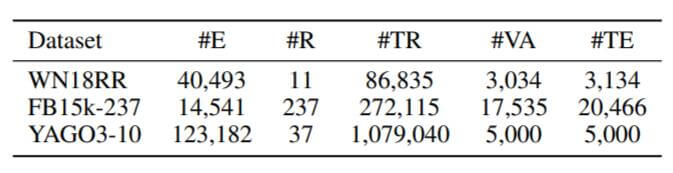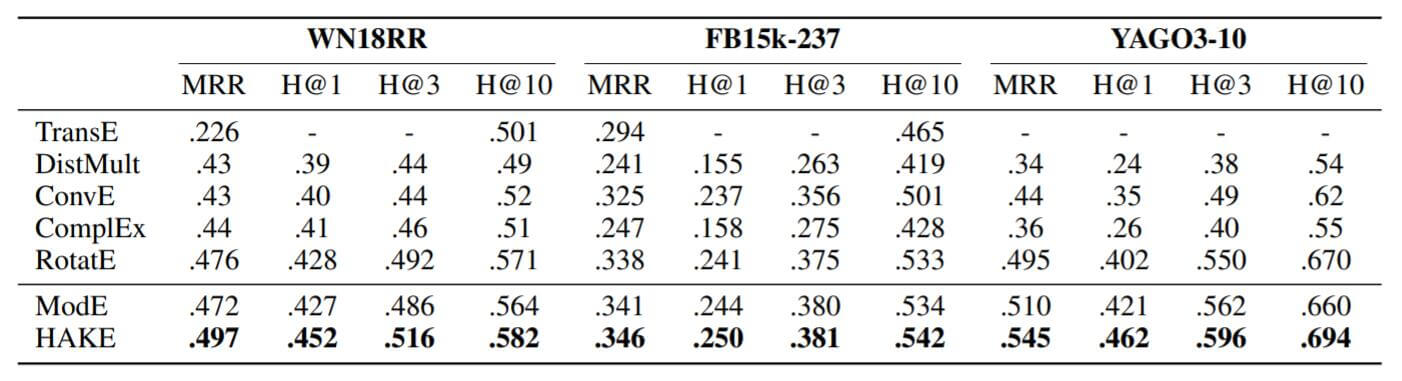HAKE将相较于RotatE有一些提升, ModE和RotatE差不多.

### Relation Embeddings Analysis

#### Modulus Part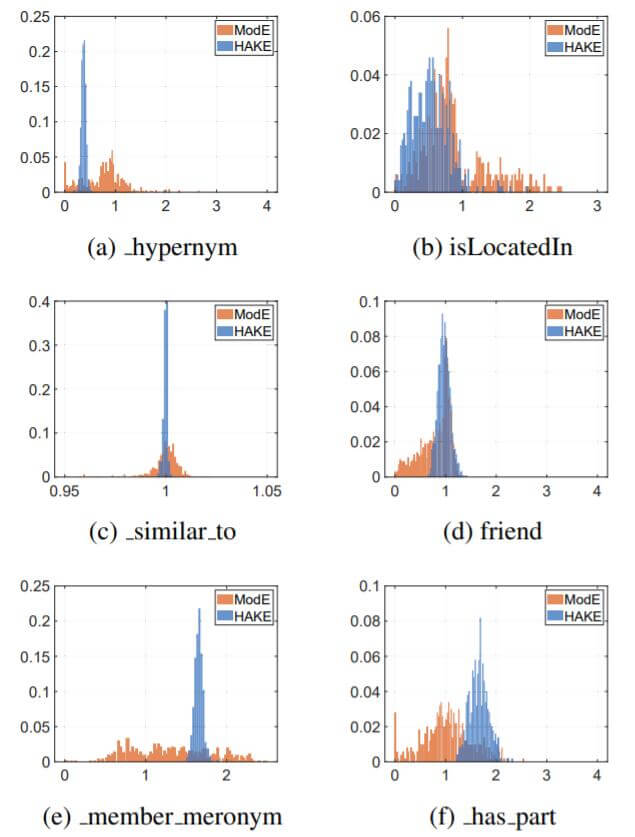• 头实体相比于尾实体的语义层次更时, 关系的模长$\mathbf{r}_{m}=\mathbf{t}_{m} / \mathbf{h}_{m}>1$.
• 头实体相比于尾实体的语义层次更时, 关系的模长$\mathbf{r}_{m}=\mathbf{t}_{m} / \mathbf{h}_{m}<1$.
• 头实体与尾实体位于相同的语义层次时, 关系的模长$\mathbf{r}_{m}=\mathbf{t}_{m} / \mathbf{h}_{m} \approx 1$.

#### Phrase Part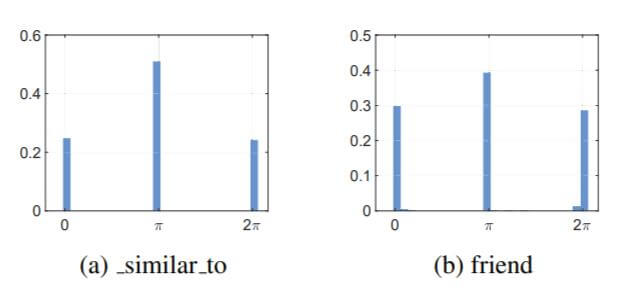### Entity Embedding Analysis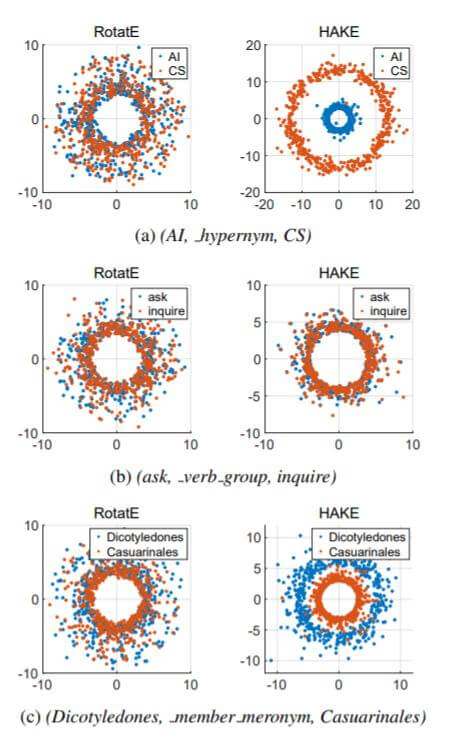### Ablation Studies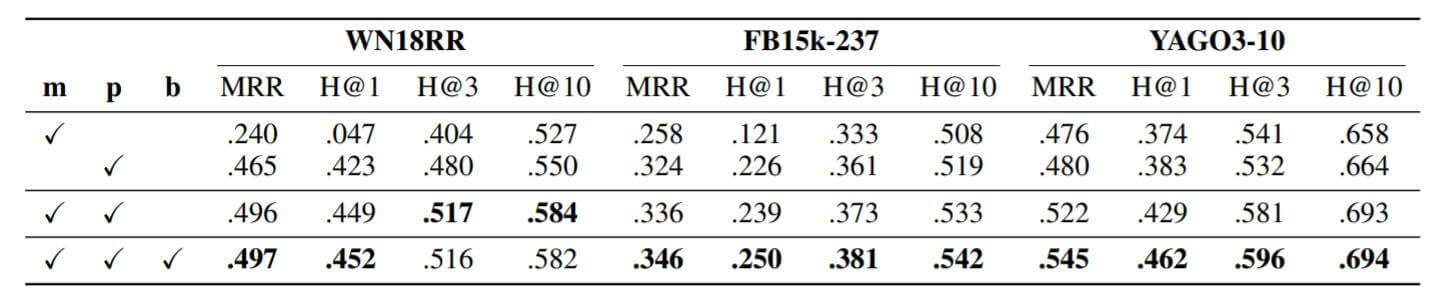$\mathbf{b}$ 代表作者所称的混合距离度量.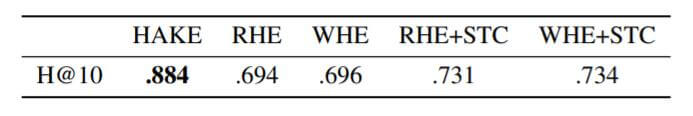## Summary

HAKE与RotatE相似, RotatE利用了复数空间的旋转建模关系, HAKE利用极坐标中的角度模长建模语义层级结构.

HAKE也十分的简洁, 但和RotatE一样, 理论上它还是只能做一对一的建模, 不善于处理多对一, 一对多, 多对多的多元关系.

上一篇GAKE: Graph Aware Knowledge Embedding
GAKE: Graph Aware Knowledge Embedding本文是论文GAKE: Graph Aware Knowledge Embedding的阅读笔记和个人理解. Basic Idea在现有的KGE方法中, 都是基于三元组
2021-04-18

目录